# Please give answer with the details. Thanks a lot! Let T: V-W be a linear transformation between vector spaces V and W...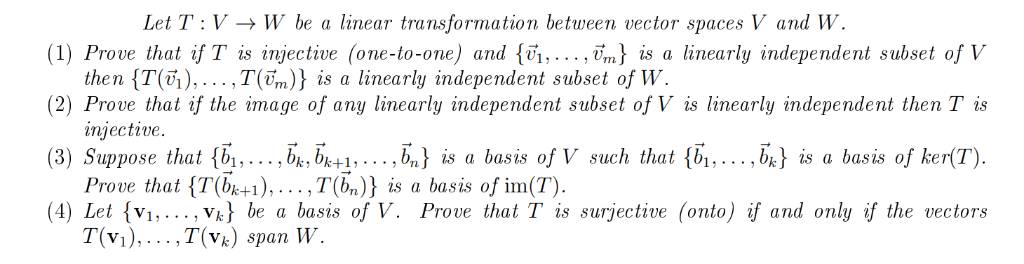Let T: V-W be a linear transformation between vector spaces V and W (1) Prove that if T is injective (one-to-one) and {vi,.. ., vm) is a linearly independent subset of V the n {T(6),…,T(ền)} is a linearly independent subset of W (2) Prove that if the image of any linearly independent subset of V is linearly independent then Tis injective. (3) Suppose that {b1,... bkbk+1,. . . ,b,) is a basis of V such that ,bk^ is a basis of ker(T). Prove that Tbk+1), , T(%)} is a basis of im(T) (4) Let v, ..., Vk) be a basis of V. Prove that T is surjective (onto) if and only if the vectors T(v) ,T(%) span W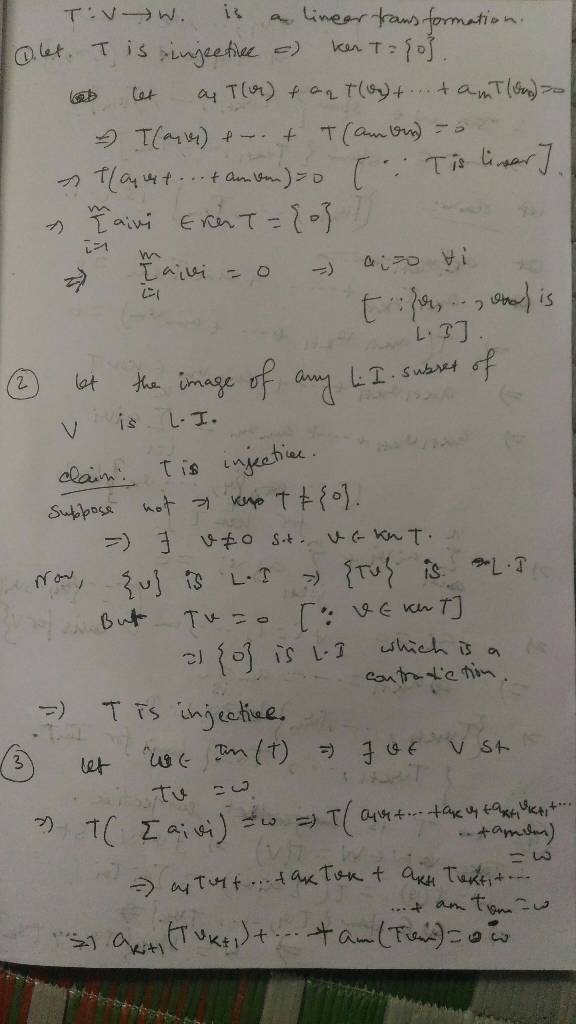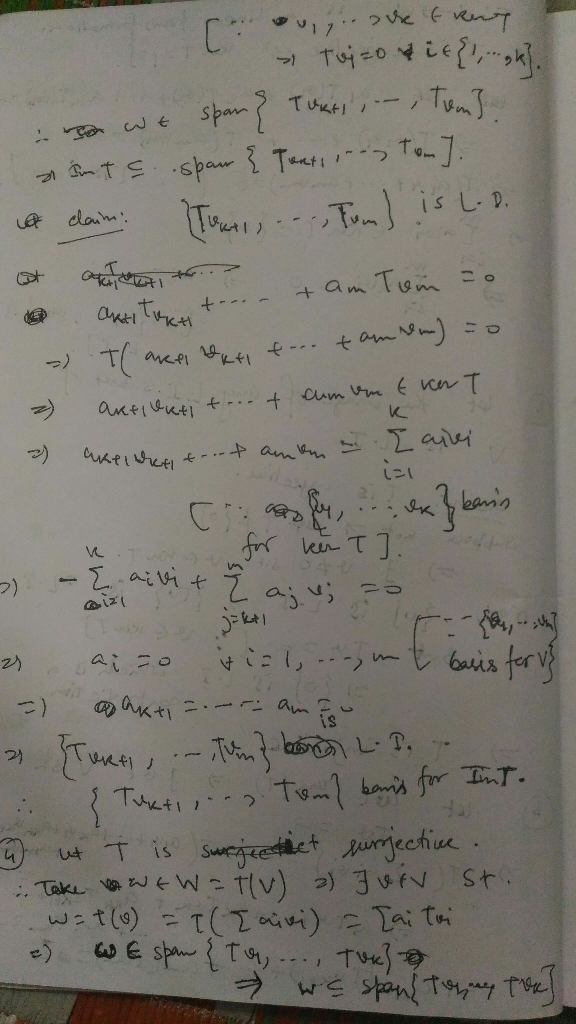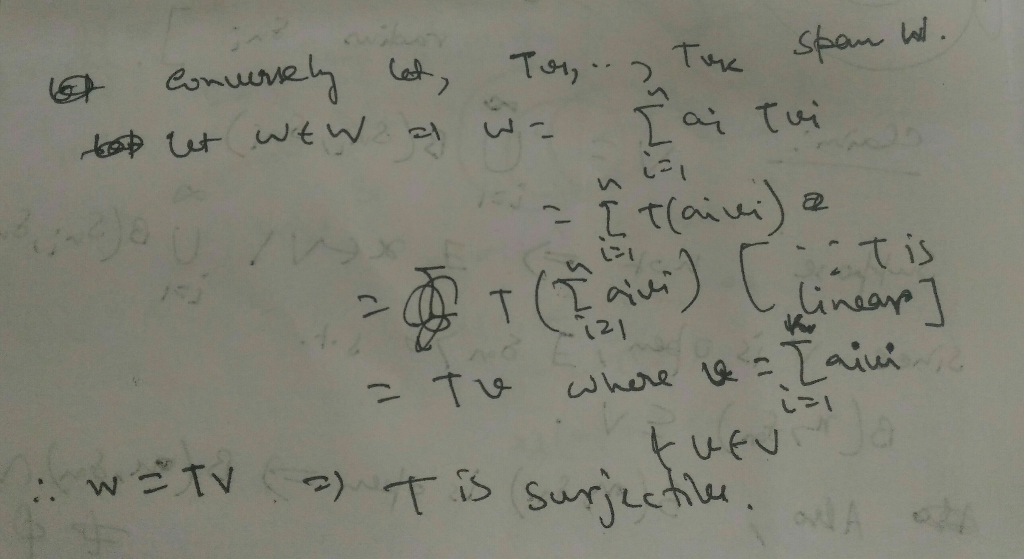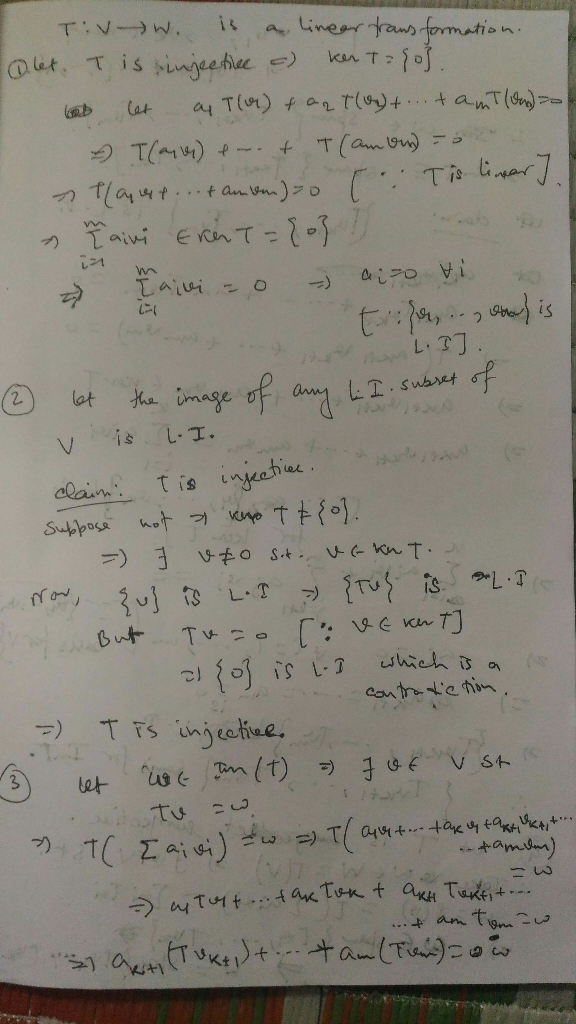##### Add Answer of: Please give answer with the details. Thanks a lot! Let T: V-W be a linear transformation between vector spaces V and W...
Similar Homework Help Questions
• ### Proble m 3. Let T: V ->W be (1) Prove that if T is then T(),... ,T(Fm)} is a linearly indepen dent subset of W (2) P...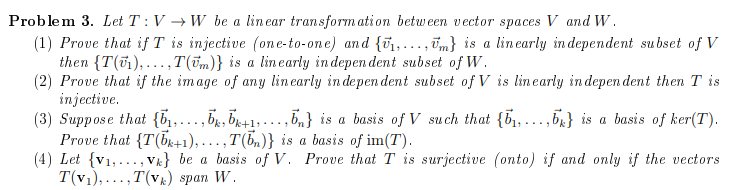Proble m 3. Let T: V ->W be (1) Prove that if T is then T(),... ,T(Fm)} is a linearly indepen dent subset of W (2) Prove that if the image of any linearly in depen dent subset of V is linearly indepen dent then T is injective (3) Suppose that {,... ,b,b^1,...,5} is Prove that T(b1), .. . , T(b,)} is a basis of im(T) (4) Let v1,. Vk} be T(v1),..,T(vk) span W lin ear transform ation between vector...

• ### Please answer me fully with the details. Thanks! True of False? Justify yo ur answer. —D т. If {ii, .., in} is a linear...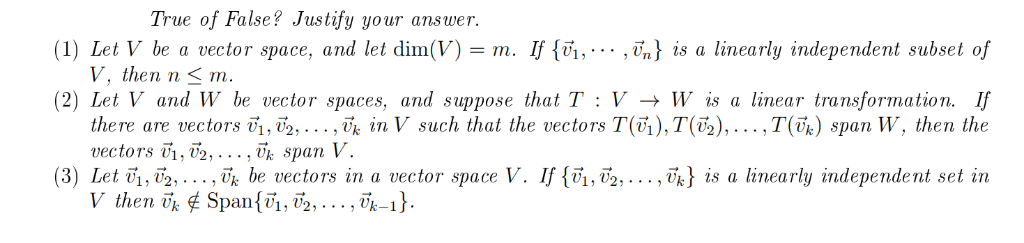Please answer me fully with the details. Thanks! True of False? Justify yo ur answer. —D т. If {ii, .., in} is a linearly independent subset of (1) Let V bea vector spacе, аnd let dim(V) V. then n < т. (2) Let V and W be vector spaces, and suppose that T : V -+ W is a linear transformation. If there are vectors i, 2, ..., Tj in V such that the vectors T(),T(T2),...,T(vj) span W, then the...

• ### Problem 5: Let V and W be vector spaces and let B = {V1, V2, ...,...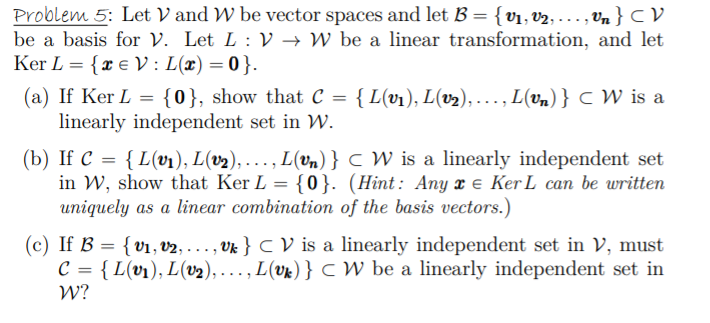Problem 5: Let V and W be vector spaces and let B = {V1, V2, ..., Un} CV be a basis for V. Let L :V + W be a linear transformation, and let Ker L = {2 € V: L(x)=0}. (a) If Ker L = {0}, show that C = {L(v1), L(02), ..., L(vn) } CW is a linearly independent set in W. (b) If C = {L(01), L(V2),..., L(Un)} C W is a linearly independent set in W,...

• ### Problem 5. Let W and U be finite-dimensional vector spaces, and let T : W > W and S : W -> U be linear transforma...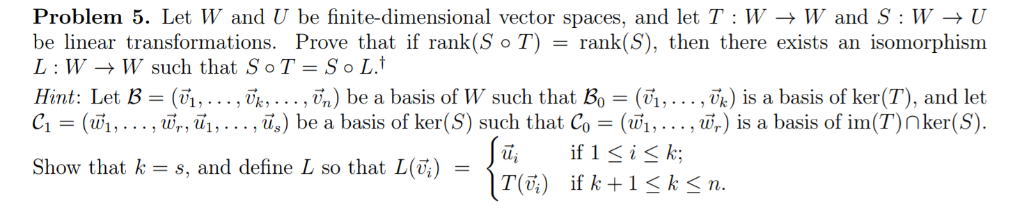Problem 5. Let W and U be finite-dimensional vector spaces, and let T : W > W and S : W -> U be linear transformations. Prove that if rank(S o T) L W W such that S o T = So L. = rank(S), then there exists an isomorphism (,.. . , Vk) is a basis of ker(T), and let (w1, ., wr) is a basis of im(T) nker(S) if 1 ik Hint: Let B (vi,... , Vk,...,vj,) be...

• ### Problem 6. Let V, W, and U be finite-dimensional vector spaces, and let T : V → W and S : W → U be linear transformatio...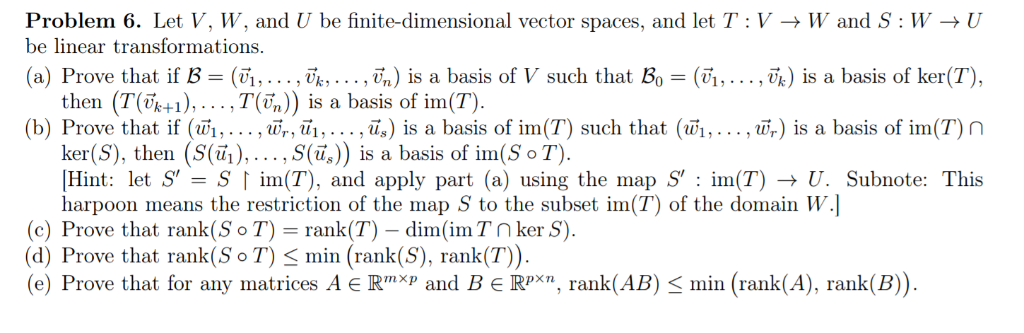Problem 6. Let V, W, and U be finite-dimensional vector spaces, and let T : V → W and S : W → U be linear transformations (a) Prove that if B-(Un . . . , v. . . . ,6) is a basis of V such that Bo-(Un .. . ,%) s a basis of ker(T) then (T(Fk+), , T(n)) is a basis of im(T) (b) Prove that if (w!, . . . ,u-, υ, . . . ,i)...

• ### Please answer me fully with the details. Thanks! Let V and W be vector spaces, let B = (j,...,Tn) be a basis of V, and...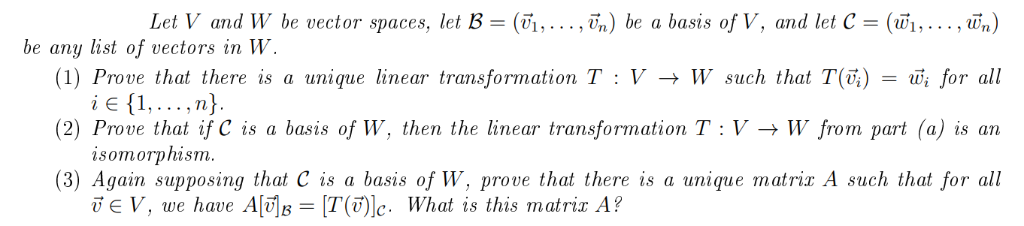Please answer me fully with the details. Thanks! Let V and W be vector spaces, let B = (j,...,Tn) be a basis of V, and let C = (Wj,..., Wn) be any list of vectors in W. (1) Prove that there is a unique linear transformation T : V -> W such that T(V;) i E 1,... ,n} (2) Prove that if C is a basis of W, then the linear transformation T : V -> W from part (a)...

• ### please be include all the details thanks In exercises 25 and 26, let V be a...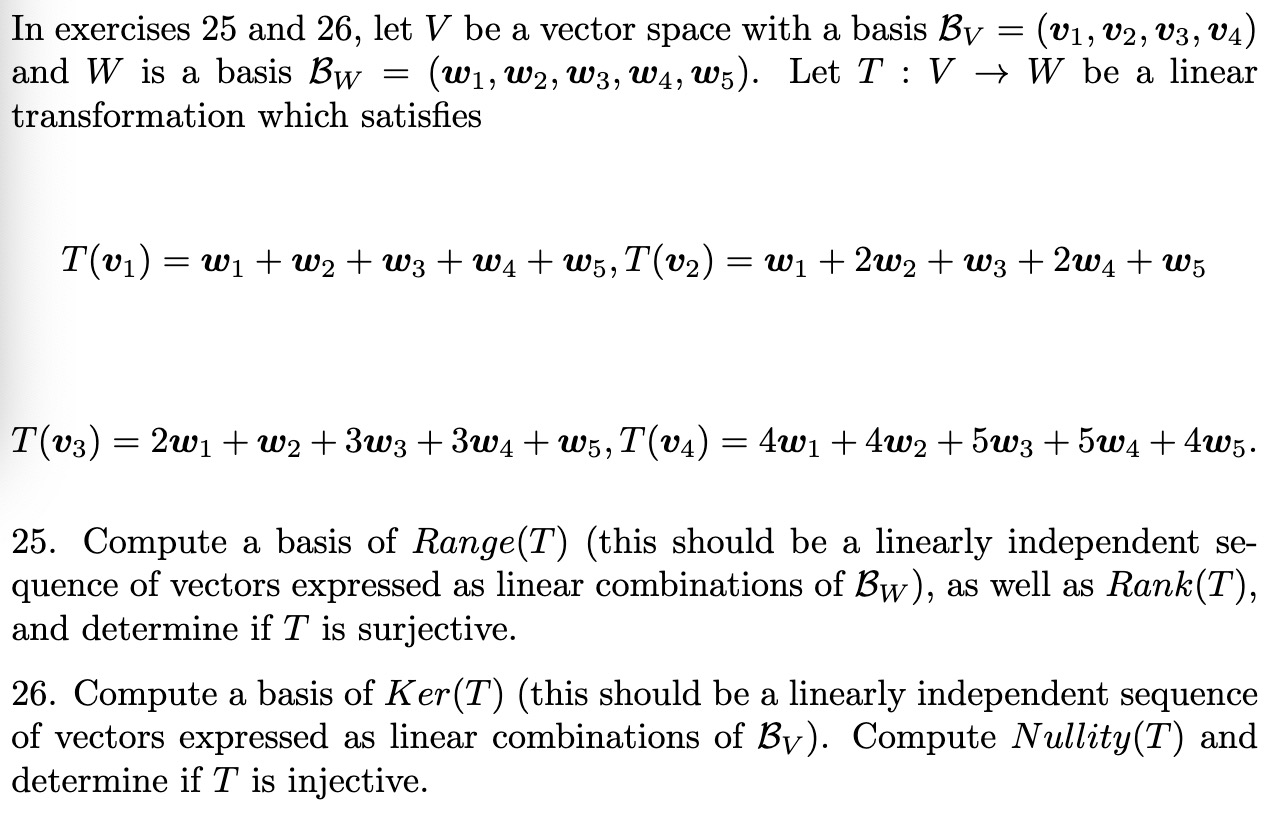please be include all the details thanks In exercises 25 and 26, let V be a vector space with a basis Bv = (v1, V2, V3, V4) and W is a basis Bw = (W1, W2, W3, W4, W5). Let T :V + W be a linear transformation which satisfies T(v1) = Wi+w2 + W3 + W4+ W5,T(v2) = W1 + 2w2 + W3 +264 + W5 T(03) = 2w1 + W2 +373 +374 + W5, T(04) = 4w1 +...

• ### Q10 10 Points Please answer the below questions. Q10.1 4 Points Let m, n EN\{1}, V...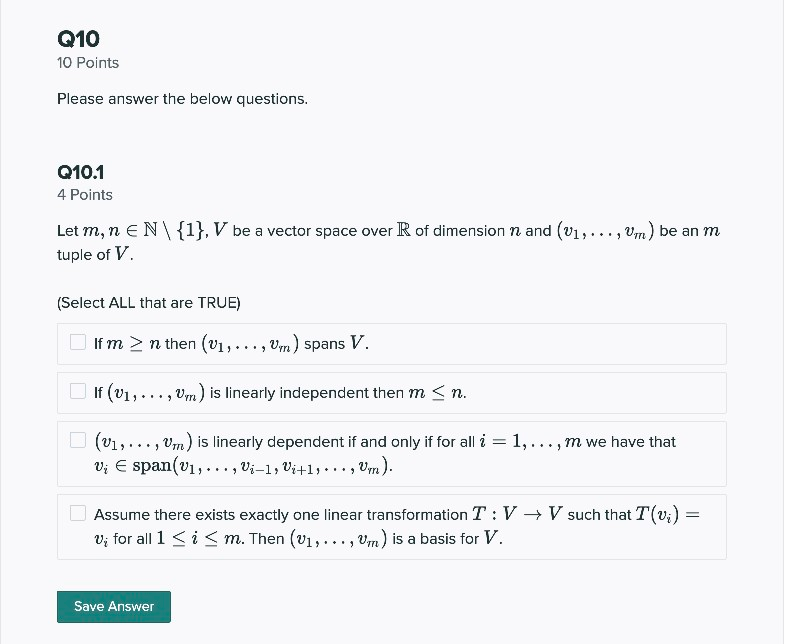Q10 10 Points Please answer the below questions. Q10.1 4 Points Let m, n EN\{1}, V be a vector space over R of dimension n and (v1,..., Vm) be an m tuple of V. (Select ALL that are TRUE) If m > n then (v1, ..., Vy) spans V. If (01,..., Vm) is linearly independent then m <n. (V1,..., Um) is linearly dependent if and only if for all i = 1,..., m we have that Vi Espan(v1,..., Vi-1, Vi+1,...,...

• ### transformation

2. Let V and W be vector spaces and T: V－>W be a linear transformation. For a given U subset of V,we denote byT(U)the subset of W deined byT(U)= {T(u)|uε U}.(a) Prove that T is one-to-one if and only if for every linearly independent subset U of V, the subsetT(U) of W is linearly independent.(b) Assume that V={v1,...,vn} is a basis for V and that T is one-to-one and onto. Prove that T(V) is a basis for W.c) Assume that...

• ### suppose that s=(v1,v2,......vm) is a finite set of linearly independent vectors in V, and w ∈ V some other vector. Let T= S ∪ (W). Prove that T is not linearly independent if and only if w∈ span(s).

suppose that s=(v1,v2,......vm) is a finite set of linearly independent vectors in V, and w ∈ V some other vector. Let T= S ∪ (W). Prove that T is not linearly independent if and only if w∈ span(s).

Free Homework App